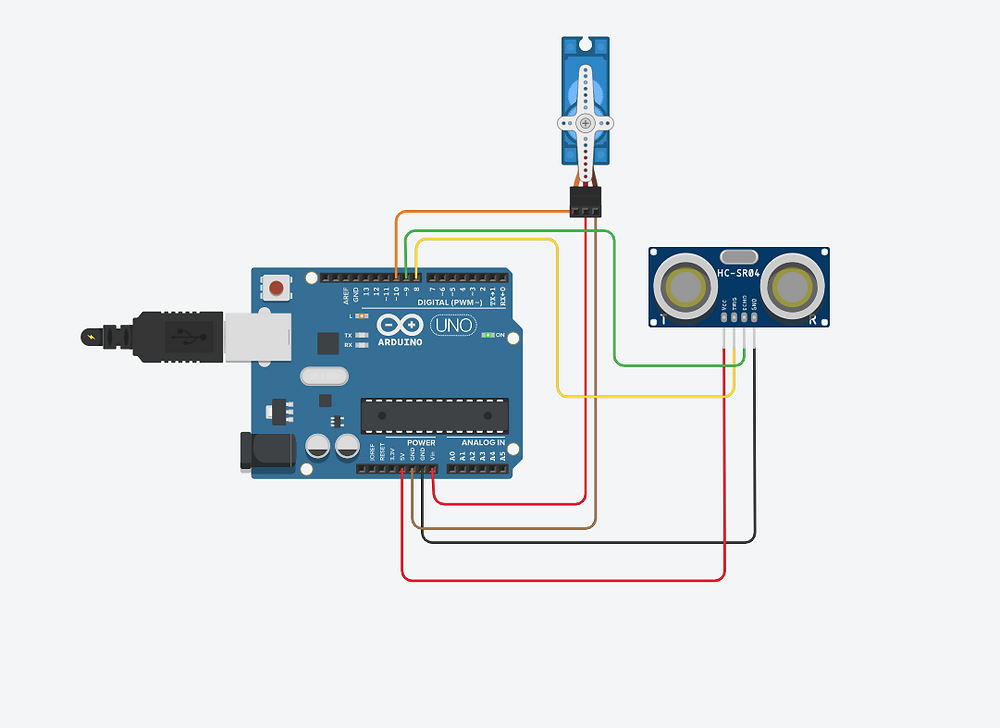Search
•Harman Hack World Tips & Tricks

# How To #Make A #Radar Using #Arduino And #Ultrasonic #Sensor Easily At #Home

Updated: Aug 10, 2020

This video all about making a project using Arduino and it's components to imitate a working prototype of radar system used in various places for ex. in airplanes, defense areas, etc. This project does not require that much of high knowledge of Arduino coding and everything.

You can easily make this project if you have these following given components:

1. Arduino Uno R3..................Amazon

3. Jumper Wires.......................Amazon

4. Servo motor...........................Amazon

5. Ultrasonic Sensor (HC-SR04).........Amazon

6. Hot glue gun.................................Amazon

(Disclosure: These are affiliate links. As an Amazon Associate I earn from qualifying purchases.)

## Circuit Diagram:Now we need to make a code and upload it to the Arduino Board that will enable the interaction between the Arduino and the Processing IDE.

Here’s the Arduino Source Code with a description of each line of the code:

// Includes the Servo library

#include <Servo.h>.

// Defines Tirg and Echo pins of the Ultrasonic Sensor

const int trigPin = 10;

const int echoPin = 11;

// Variables for the duration and the distance

long duration;

int distance;

Servo myServo; // Creates a servo object for controlling the servo motor

void setup() {

pinMode(trigPin, OUTPUT); // Sets the trigPin as an Output

pinMode(echoPin, INPUT); // Sets the echoPin as an Input

Serial.begin(9600);

myServo.attach(12); // Defines on which pin is the servo motor attached

}

void loop() {

// rotates the servo motor from 15 to 165 degrees

for(int i=15;i<=165;i++){

myServo.write(i);

delay(30);

distance = calculateDistance();// Calls a function for calculating the distance measured by the Ultrasonic sensor for each degree

Serial.print(i); // Sends the current degree into the Serial Port

Serial.print(","); // Sends addition character right next to the previous value needed later in the Processing IDE for indexing

Serial.print(distance); // Sends the distance value into the Serial Port

Serial.print("."); // Sends addition character right next to the previous value needed later in the Processing IDE for indexing

}

// Repeats the previous lines from 165 to 15 degrees

for(int i=165;i>15;i--){

myServo.write(i);

delay(30);

distance = calculateDistance();

Serial.print(i);

Serial.print(",");

Serial.print(distance);

Serial.print(".");

}

}

// Function for calculating the distance measured by the Ultrasonic sensor

int calculateDistance(){

digitalWrite(trigPin, LOW);

delayMicroseconds(2);

// Sets the trigPin on HIGH state for 10 micro seconds

digitalWrite(trigPin, HIGH);

delayMicroseconds(10);

digitalWrite(trigPin, LOW);

duration = pulseIn(echoPin, HIGH); // Reads the echoPin, returns the sound wave travel time in microseconds

distance= duration*0.034/2;

return distance;

}

Here’s the complete Processing Source Code of the Arduino Radar:

import processing.serial.*; // imports library for serial communication

import java.awt.event.KeyEvent; // imports library for reading the data from the serial port

import java.io.IOException;

Serial myPort; // defines Object Serial

// defubes variables

String angle="";

String distance="";

String data="";

String noObject;

float pixsDistance;

int iAngle, iDistance;

int index1=0;

int index2=0;

PFont orcFont;

void setup() {

size (1200, 700); // ***CHANGE THIS TO YOUR SCREEN RESOLUTION***

smooth();

myPort = new Serial(this,"COM5", 9600); // starts the serial communication

myPort.bufferUntil('.'); // reads the data from the serial port up to the character '.'. So actually it reads this: angle,distance.

}

void draw() {

fill(98,245,31);

// simulating motion blur and slow fade of the moving line

noStroke();

fill(0,4);

rect(0, 0, width, height-height*0.065);

fill(98,245,31); // green color

// calls the functions for drawing the radar

drawLine();

drawObject();

drawText();

}

void serialEvent (Serial myPort) { // starts reading data from the Serial Port

// reads the data from the Serial Port up to the character '.' and puts it into the String variable "data".

data = data.substring(0,data.length()-1);

index1 = data.indexOf(","); // find the character ',' and puts it into the variable "index1"

angle= data.substring(0, index1); // read the data from position "0" to position of the variable index1 or thats the value of the angle the Arduino Board sent into the Serial Port

distance= data.substring(index1+1, data.length()); // read the data from position "index1" to the end of the data pr thats the value of the distance

// converts the String variables into Integer

iAngle = int(angle);

iDistance = int(distance);

}

pushMatrix();

translate(width/2,height-height*0.074); // moves the starting coordinats to new location

noFill();

strokeWeight(2);

stroke(98,245,31);

// draws the arc lines

arc(0,0,(width-width*0.0625),(width-width*0.0625),PI,TWO_PI);

arc(0,0,(width-width*0.27),(width-width*0.27),PI,TWO_PI);

arc(0,0,(width-width*0.479),(width-width*0.479),PI,TWO_PI);

arc(0,0,(width-width*0.687),(width-width*0.687),PI,TWO_PI);

// draws the angle lines

line(-width/2,0,width/2,0);

popMatrix();

}

void drawObject() {

pushMatrix();

translate(width/2,height-height*0.074); // moves the starting coordinats to new location

strokeWeight(9);

stroke(255,10,10); // red color

pixsDistance = iDistance*((height-height*0.1666)*0.025); // covers the distance from the sensor from cm to pixels

// limiting the range to 40 cms

if(iDistance<40){

// draws the object according to the angle and the distance

}

popMatrix();

}

void drawLine() {

pushMatrix();

strokeWeight(9);

stroke(30,250,60);

translate(width/2,height-height*0.074); // moves the starting coordinats to new location

popMatrix();

}

void drawText() { // draws the texts on the screen

pushMatrix();

if(iDistance>40) {

noObject = "Out of Range";

}

else {

noObject = "In Range";

}

fill(0,0,0);

noStroke();

rect(0, height-height*0.0648, width, height);

fill(98,245,31);

textSize(25);

text("10cm",width-width*0.3854,height-height*0.0833);

text("20cm",width-width*0.281,height-height*0.0833);

text("30cm",width-width*0.177,height-height*0.0833);

text("40cm",width-width*0.0729,height-height*0.0833);

textSize(40);

text("Indian Lifehacker ", width-width*0.875, height-height*0.0277);

text("Angle: " + iAngle +" °", width-width*0.48, height-height*0.0277);

text("Distance: ", width-width*0.26, height-height*0.0277);

if(iDistance<40) {

text(" " + iDistance +" cm", width-width*0.225, height-height*0.0277);

}

textSize(25);

fill(98,245,60);

text("30°",0,0);

resetMatrix();

text("60°",0,0);

resetMatrix();

text("90°",0,0);

resetMatrix();

text("120°",0,0);

resetMatrix();

text("150°",0,0);

popMatrix();

}